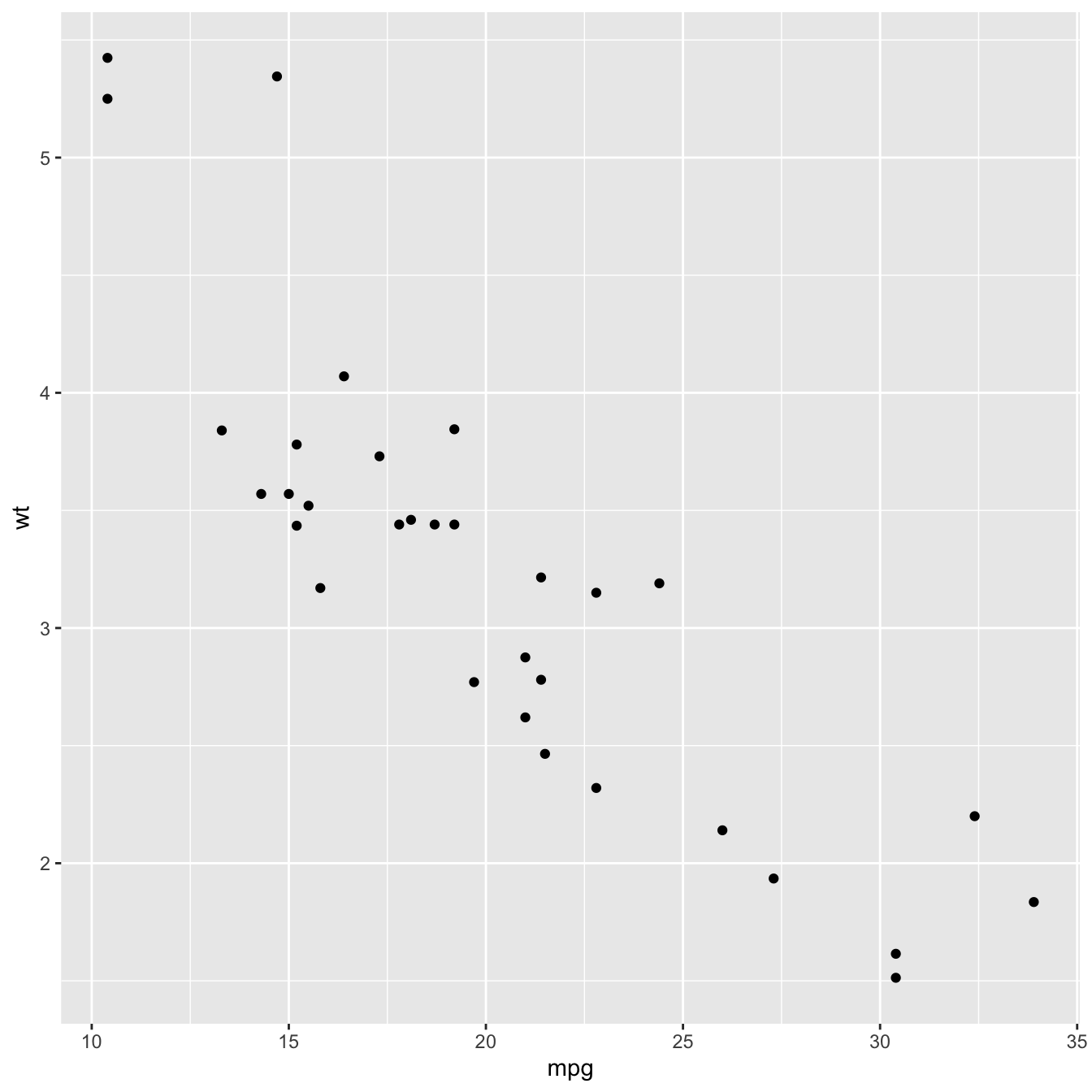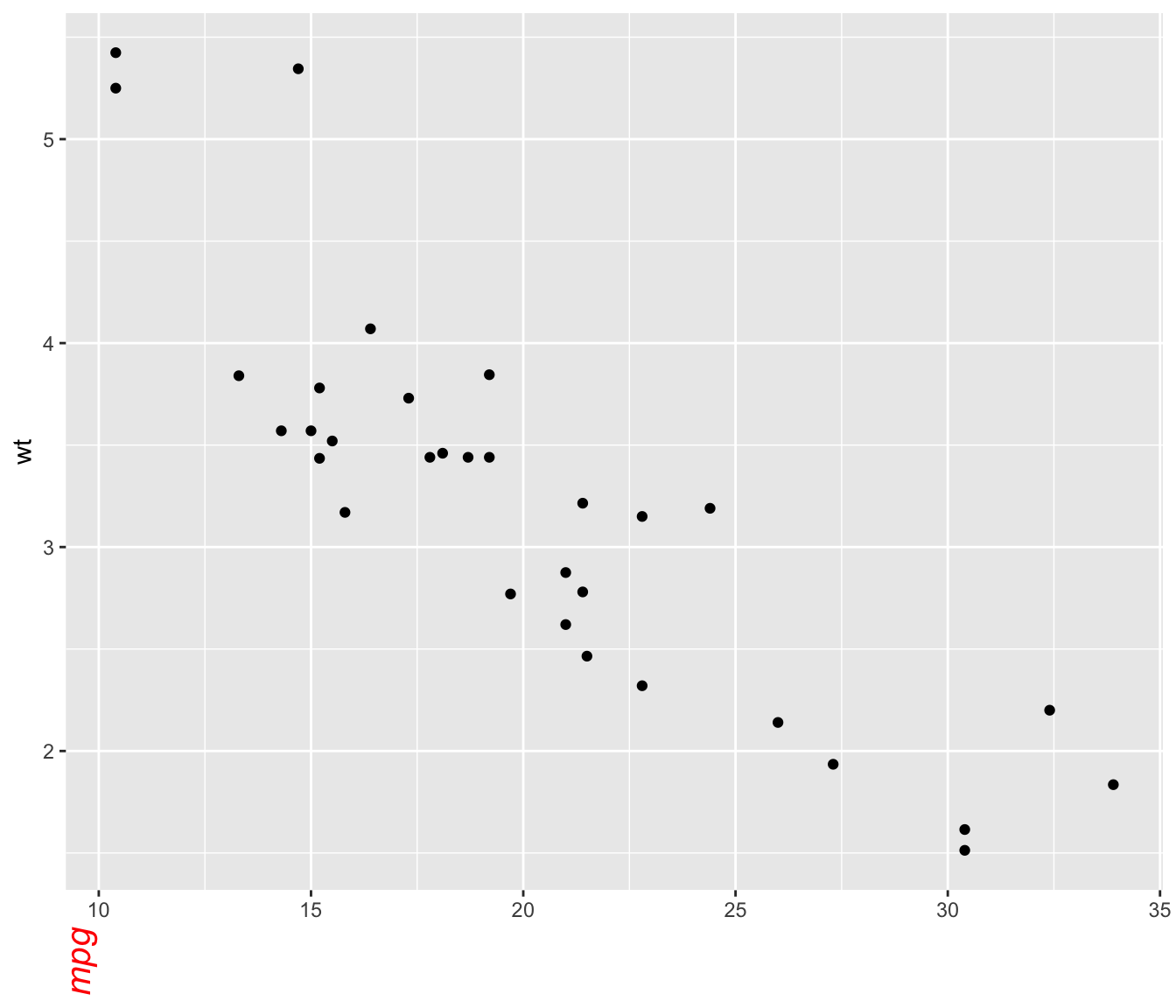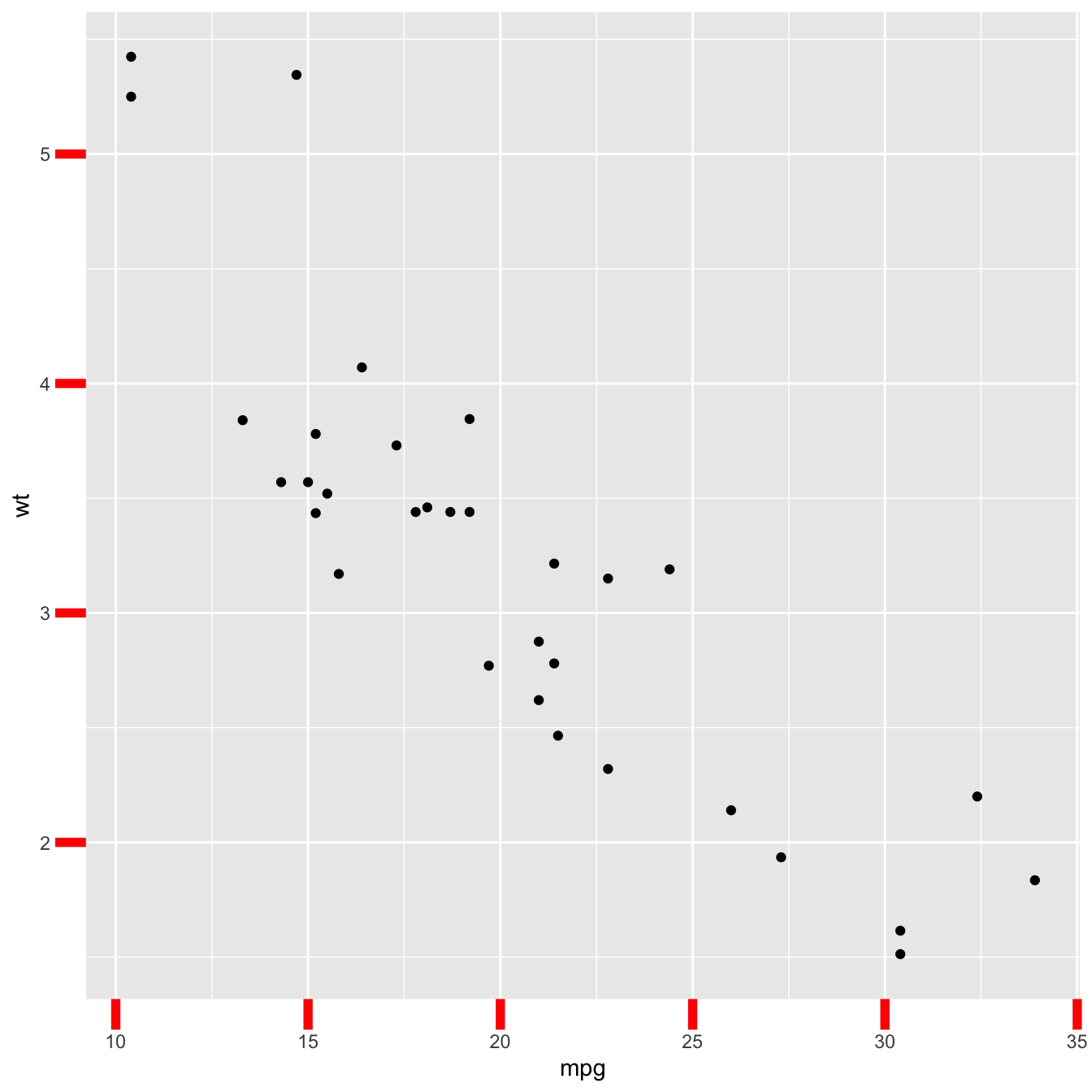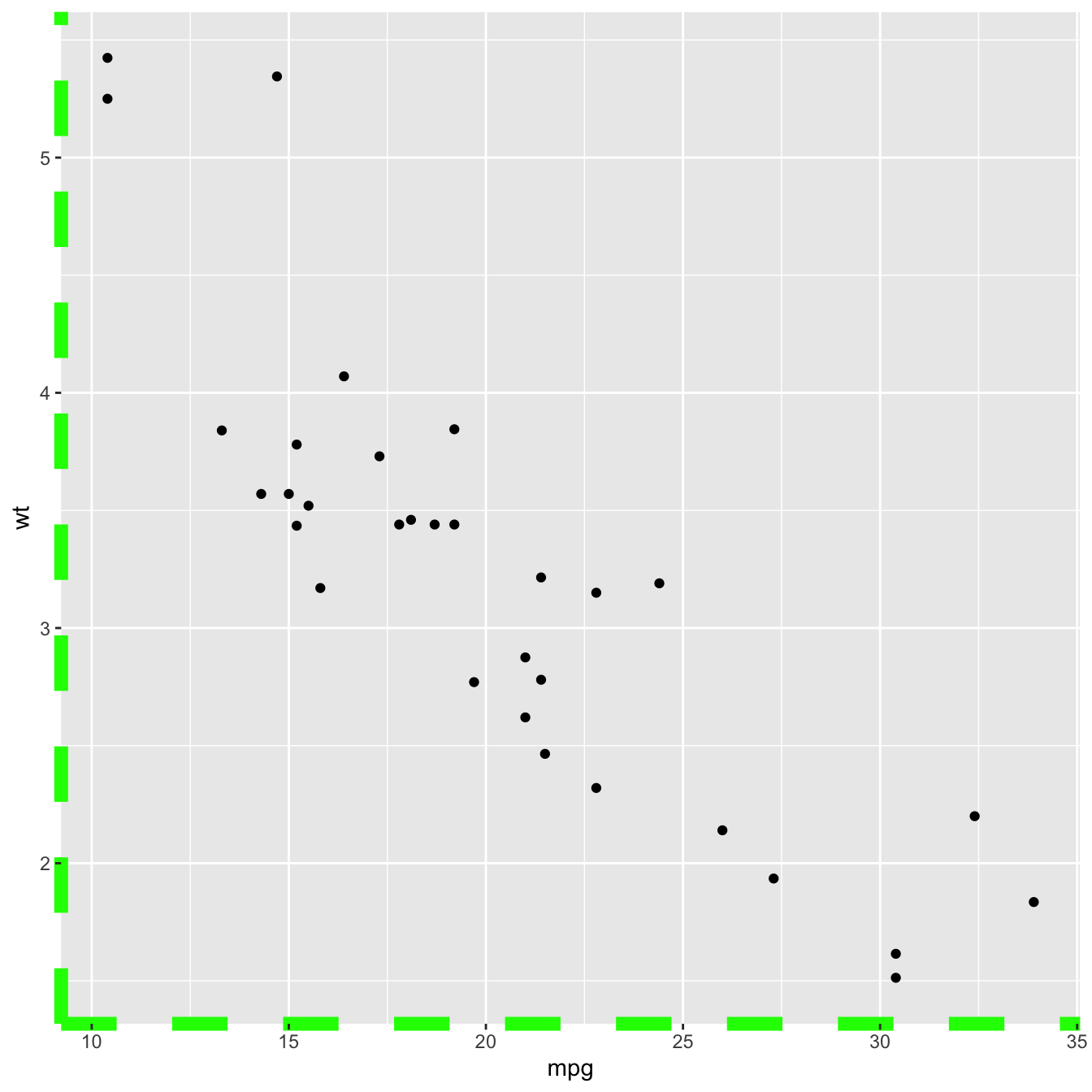# Axis manipulation with R and ggplot2

This post describes all the available options to customize chart axis with `R` and `ggplot2`. It shows how to control the axis itself, its label, title, position and more.

# Default `ggplot2` axis

Let’s start with a very basic `ggplot2` scatterplot. The axis usually looks very good with default option as you can see here.

Basically two main functions will allow to customize it:

• `theme()` to change the axis appearance
• `scale_x_` and `scale_y_` to change the axis type

Let’s see how to use them``````# Load ggplot2
library(ggplot2)

# Very basic chart
basic <- ggplot( mtcars , aes(x=mpg, y=wt)) +
geom_point()
basic``````

# Set axis title and limits with `xlab()` and `xlim()`

Two basic options that are used in almost every charts are `xlab()` and `xlim()` to control the axis title and the axis limits respectively.

Note : it is possible to specify only the lower or upper bound of a limit. For instance, try `xlim(0,NA)```````basic+
xlab("mpg value") +
xlim(0,50)``````

# Customize axis title appearance: `axis.title`The `theme()` function allows to customize all parts of the `ggplot2` chart. The `axis.title.` controls the axis title appearance. Since it is text, features are wrapped in a `element_text()` function. The code below shows how to change the most common features:

``````# Left -> both axis are modified
basic + theme(axis.title = element_text( angle = 90, color="red", size=15, face=3)) # face = title location``````
``````# Right -> only the x axis is modified
basic + theme(axis.title.x = element_text( angle = 90, color="red", size=15, face=3))``````

# Customize axis labels: `axis.text`

Using pretty much the same process, the `axis.text()` function allows to control text label features. Once more, using `axis.text.x()` would modify the x axis only.``````basic +
theme(axis.text = element_text(
angle = 90,
color="blue",
size=15,
face=3)
)``````

# Customize axis ticks and axis line with `axis.ticks` and `axis.line`The `axis.ticks()` function controls the ticks appearance. `axis.line()` controles the axis line. Both of them are lines, so options are wrapped in a `element_line()` statement.

`linetype` controls the type of line in use, see the ggplot2 section for more.

``````# chart 1: ticks
basic + theme(
axis.ticks = element_line(size = 2, color="red") ,
axis.ticks.length = unit(.5, "cm")
)``````
``````# chart 2: axis lines
basic + theme(axis.line = element_line(size = 3, colour = "green", linetype=2))``````
``````# chart 3: combination
ggplot( mtcars , aes(x=mpg, y=wt)) + geom_point() +
theme(
axis.title = element_text( color="red", size=15, face=2),
axis.line = element_line(size = 3, colour = "green", linetype=2),
axis.text = element_text( angle = 90, color="blue", size=15, face=2)
)``````

Related chart types

## Contact

This document is a work by Yan Holtz. Any feedback is highly encouraged. You can fill an issue on Github, drop me a message on Twitter, or send an email pasting yan.holtz.data with gmail.com.